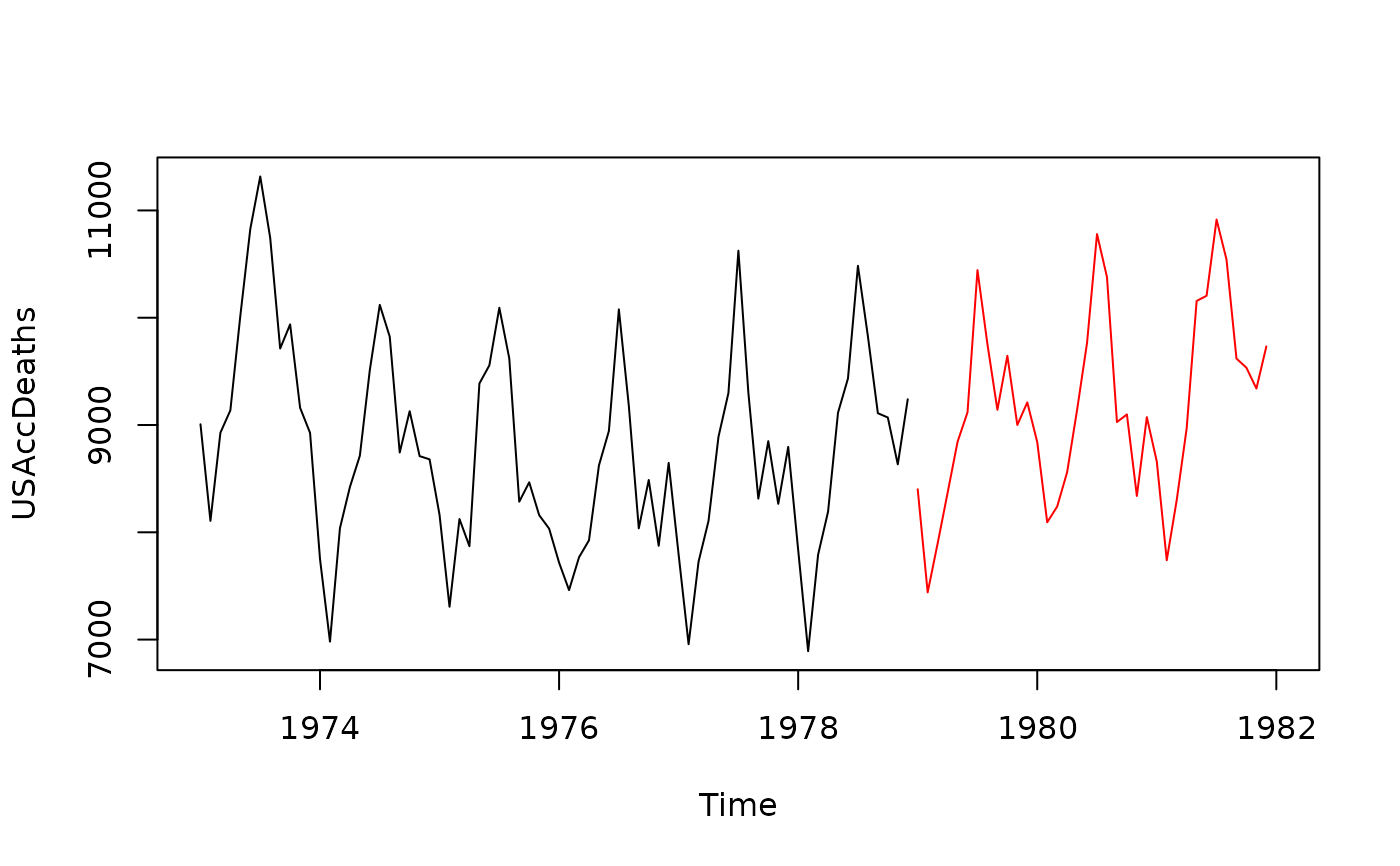Returns a time series based on the model object object.

# S3 method for ets
simulate(
object,
nsim = length(object$x), seed = NULL, future = TRUE, bootstrap = FALSE, innov = NULL, ... ) # S3 method for Arima simulate( object, nsim = length(object$x),
seed = NULL,
xreg = NULL,
future = TRUE,
bootstrap = FALSE,
innov = NULL,
lambda = object$lambda, ... ) # S3 method for ar simulate( object, nsim = object$n.used,
seed = NULL,
future = TRUE,
bootstrap = FALSE,
innov = NULL,
...
)

# S3 method for lagwalk
simulate(
object,
nsim = length(object$x), seed = NULL, future = TRUE, bootstrap = FALSE, innov = NULL, lambda = object$lambda,
...
)

# S3 method for fracdiff
simulate(
object,
nsim = object$n, seed = NULL, future = TRUE, bootstrap = FALSE, innov = NULL, ... ) # S3 method for nnetar simulate( object, nsim = length(object$x),
seed = NULL,
xreg = NULL,
future = TRUE,
bootstrap = FALSE,
innov = NULL,
lambda = object$lambda, ... ) # S3 method for modelAR simulate( object, nsim = length(object$x),
seed = NULL,
xreg = NULL,
future = TRUE,
bootstrap = FALSE,
innov = NULL,
lambda = object\$lambda,
...
)

## Arguments

object An object of class "ets", "Arima", "ar" or "nnetar". Number of periods for the simulated series. Ignored if either xreg or innov are not NULL. Either NULL or an integer that will be used in a call to set.seed before simulating the time series. The default, NULL, will not change the random generator state. Produce sample paths that are future to and conditional on the data in object. Otherwise simulate unconditionally. Do simulation using resampled errors rather than normally distributed errors or errors provided as innov. A vector of innovations to use as the error series. Ignored if bootstrap==TRUE. If not NULL, the value of nsim is set to length of innov. Other arguments, not currently used. New values of xreg to be used for forecasting. The value of nsim is set to the number of rows of xreg if it is not NULL. Box-Cox transformation parameter. If lambda="auto", then a transformation is automatically selected using BoxCox.lambda. The transformation is ignored if NULL. Otherwise, data transformed before model is estimated.

## Value

An object of class "ts".

## Details

With simulate.Arima(), the object should be produced by Arima or auto.arima, rather than arima. By default, the error series is assumed normally distributed and generated using rnorm. If innov is present, it is used instead. If bootstrap=TRUE and innov=NULL, the residuals are resampled instead.

When future=TRUE, the sample paths are conditional on the data. When future=FALSE and the model is stationary, the sample paths do not depend on the data at all. When future=FALSE and the model is non-stationary, the location of the sample paths is arbitrary, so they all start at the value of the first observation.

ets, Arima, auto.arima, ar, arfima, nnetar.

Rob J Hyndman

## Examples

fit <- ets(USAccDeaths)
plot(USAccDeaths, xlim=c(1973,1982))
lines(simulate(fit, 36), col="red")